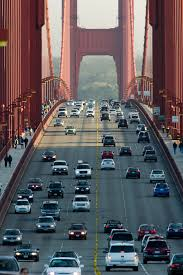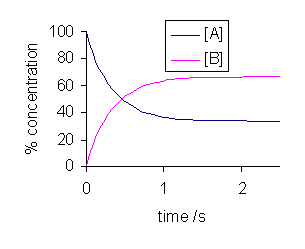## Equilibrium

#### Learning Objective

• Recall the relationship between the forward and reverse reaction rates when a reaction is at equilibrium

#### Key Points

• In a chemical equilibrium, the forward and reverse reactions occur at equal rates, and the concentrations of products and reactants remain constant.
• A catalyst speeds up the rate of a chemical reaction, but has no effect upon the equilibrium position for that reaction.

#### Terms

• the equilibrium positionThe point in a chemical reaction at which the concentrations of reactants and products are no longer changing.
• chemical equilibriumIn a chemical reaction, the state in which both reactants and products are present at concentrations that have no further tendency to change with time.

In theory, all chemical reactions are in fact double reactions: for every forward reaction, there is a subsequent reverse reaction. The idea can be illustrated as follows:

$reactants\rightleftharpoons products$

For plenty of reactions, however, the forward reaction is so favored, and the reverse reaction is so negligible, that reactions are written simply in terms of the solid forward arrow, $A\rightarrow B$. However, we will now consider forward/reverse reaction pairs that exist in chemical equilibrium with one another.

## The Concept of Chemical Equilibrium

In a chemical reaction, chemical equilibrium is the state in which the forward reaction rate and the reverse reaction rate are equal. The result of this equilibrium is that the concentrations of the reactants and the products do not change. However, just because concentrations aren’t changing does not mean that all chemical reaction has ceased. Just the opposite is true; chemical equilibrium is a dynamic state in which reactants are being converted into products at all times, but at the exact rate that products are being converted back into reactants. The result of such a situation is analogous to a bridge between two cities, where the rate of cars going over the bridge in each direction is exactly equal. The result is that the net number of cars on either side of the bridge does not change.Cars entering and leaving a city over a bridgeChemical equilibrium is akin to two cities connected a bridge. If the rate at which cars leave the city is exactly equal to the rate at which cars enter the city, the result is that the net number of cars on both sides of the bridge does not change.

## Reaching Equilibrium and the Equilibrium Position

When a system consists of competing forward and reverse reaction rates, the reaction will proceed until chemical equilibrium is reached. Consider the following general reaction between A and B:

$A\rightleftharpoons B$

Let’s say that A and B are introduced into a reaction vessel, and a reaction begins to proceed. The concentrations of A and B will change over time, until they reach equilibrium.Concentration vs. time for a reversible reactionNote that equilibrium is reached when both the curves plateau, and the concentrations of both reactants and products do not change afterward.

Note that over time, the curves level out, or plateau, and the concentrations of A and B are no longer changing. This is the point at which the system has reached chemical equilibrium. While there are various factors that can increase or decrease the amount of time it takes for a given system to reach equilibrium, the equilibrium position itself is unaffected by these factors. For instance, if a catalyst is added to the system, the reaction will proceed more quickly, and equilibrium will be reached faster, but the concentrations of both A and B will be the same at equilibrium for both the catalyzed and the uncatalyzed reaction.

## Conclusion

The equilibrium state is one in which there is no net change in the concentrations of reactants and products. Despite the fact that there is no apparent change at equilibrium, this does not mean that all chemical reaction has ceased. Nothing could be further from the truth; at equilibrium, the forward and reverse reactions continue, but at identical rates, thereby leaving the net concentrations of reactants and products undisturbed.